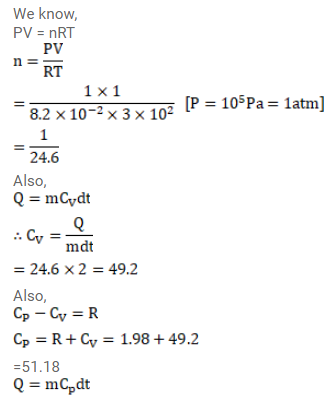# A sample of air weighing 1.18g occupiesQuestion:

A sample of air weighing $1.18 \mathrm{~g}$ occupies $1.0 \times 10^{3} \mathrm{~cm}^{3}$ when kept at $300 \mathrm{k}$ and $1.0 \times 10^{5} \mathrm{~Pa}$. When $2.0$ cal of heat is added to it at constant volume, its temperature increase the temperature of air by $1^{\circ} \mathrm{C} t$ constant pressure, if the temperature of air by $1^{\circ} \mathrm{C}$ at constant pressure, if the mechanical equivalent of heat is $4.2 \times 10^{7} \mathrm{erg} / \mathrm{cal}$. Assume that air behave as an ideal gas.

Solution:$=\frac{1}{24.6} \times 51.18 \times 1$

$=2.08 \mathrm{cal}$